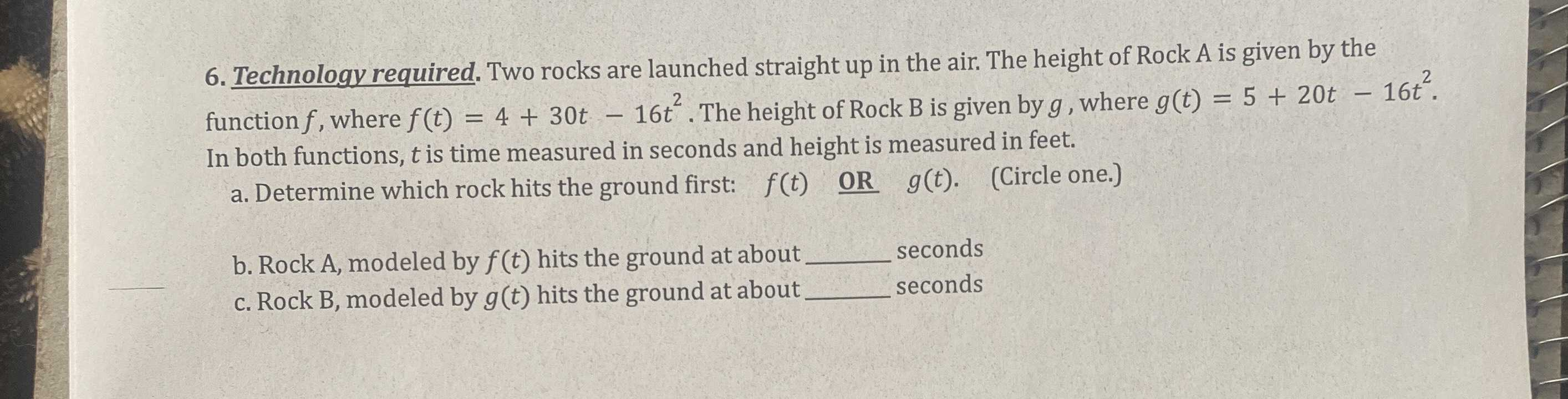### ¿Todavía tienes preguntas de matemáticas?

Pregunte a nuestros tutores expertos
Algebra
Pregunta6. Technology required. Two rocks are launched straight up in the air. The height of Rock A is given by the function $$f$$ , where $$f ( t ) = 4 + 30 t - 16 t ^ { 2 }$$ . The height of Rock B is given by $$g$$ , where $$g ( t ) = 5 + 20 t - 16 t ^ { 2 }$$ . In both functions, $$t$$ is time measured in seconds and height is measured in feet. a. Determine which rock hits the ground first: $$f ( t ) \underline {$$ oR $$} g ( t )$$ . (Circle one.) b. Rock A, modeled by $$f ( t )$$ hits the ground at about __ seconds c. Rock B, modeled by $$g ( t )$$ hits the ground at about